Home

# Existence of Multiple Solutions for a p-Laplacian System in ℝ N with Sign-changing Weight Functions

## Abstract

In this paper, we consider the quasi-linear elliptic problem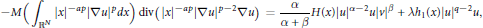$$-M\left( {{\int }_{{{\mathbb{R}}^{N}}}}{{\left| x \right|}^{-ap}}{{\left| {{\nabla }_{u}} \right|}^{p}}dx \right)\,\text{div}\left( {{\left| x \right|}^{-ap}}{{\left| \nabla u \right|}^{p-2}}\nabla u \right)=\frac{\alpha }{\alpha +\beta }H\left( x \right){{\left| u \right|}^{\alpha -2}}u{{\left| v \right|}^{\beta }}+\text{ }\lambda \text{ }{{\text{h}}_{1}}\left( x \right){{\left| u \right|}^{q-2}}u,$$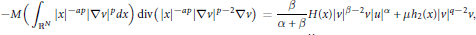$$-M\left( {{\int }_{{{\mathbb{R}}^{N}}}}{{\left| x \right|}^{-ap}}{{\left| \nabla v \right|}^{p}}dx \right)\,\text{div}\left( {{\left| x \right|}^{-ap}}{{\left| \nabla v \right|}^{p-2}}\nabla v \right)=\frac{\beta }{\alpha +\beta }H\left( x \right){{\left| v \right|}^{\beta -2}}v{{\left| u \right|}^{\alpha }}+\mu {{h}_{2}}\left( x \right){{\left| v \right|}^{q-2}}v,$$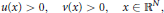$$u\left( x \right)>0,v\left( x \right)>0,x\in {{\mathbb{R}}^{N}},$$

where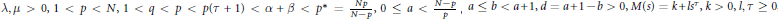$\text{ }\lambda \text{ ,}\mu >\text{0,}\text{1}<\text{p}<\text{N,}\text{1}<\text{q}<\text{p}<\text{p}\left( \tau +1 \right)<\alpha +\beta <{{p}^{*}}=\frac{{{N}_{p}}}{N-p},0\le a<\frac{N-p}{p},a\le b<a+1,d=a+1-b>0,M\left( s \right)=k+l{{s}^{\tau }},k>0,l,\tau \ge 0$ and the weight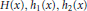$H\left( x \right),\,{{h}_{1}}\left( x \right),\,{{h}_{2}}\left( x \right)$ are continuous functions that change sign in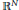${{\mathbb{R}}^{N}}$ . We will prove that the problem has at least two positive solutions by using the Nehari manifold and the fibering maps associated with the Euler functional for this problem.

## References

Hide All
MathJax
MathJax is a JavaScript display engine for mathematics. For more information see http://www.mathjax.org.

# Existence of Multiple Solutions for a p-Laplacian System in ℝ N with Sign-changing Weight Functions

## Metrics

### Full text viewsFull text views reflects the number of PDF downloads, PDFs sent to Google Drive, Dropbox and Kindle and HTML full text views.

Total number of HTML views: 0
Total number of PDF views: 0 *Loading metrics...

### Abstract viewsAbstract views reflect the number of visits to the article landing page.

Total abstract views: 0 *Loading metrics...

* Views captured on Cambridge Core between <date>. This data will be updated every 24 hours.

Usage data cannot currently be displayed